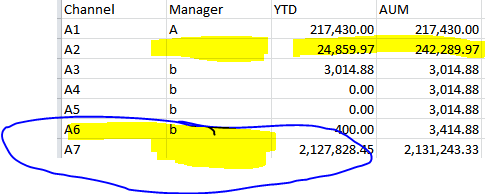# QlikView App Dev

Discussion Board for collaboration related to QlikView App Development.

Announcements
Join us at the Cloud Data and Analytics Tour! REGISTER TODAY
cancel
Showing results for
Search instead for
Did you mean:Creator III

## null value in dimension column creates problem in above() function

Hi,

Below are the sample data that I am getting

 Channel Manager YTD AUM A1 A 217,430.00 217,430.00 A2 24,859.97 242,289.97 A3 b 3,014.88 3,014.88 A4 b 0.00 3,014.88 A5 b 0.00 3,014.88 A6 b 400.00 3,414.88 A7 2,127,828.45 2,131,243.33

and below is the set expression that I have used:-

rangesum(above(sum({\$<[ProcDate]={">=\$(=YearStart(Max([ProcDate]))) <=\$(=Max([Process Date]))"}>}[Amt]),0,RowNo(TOTAL)))

to find out the accumulated value and we can see that whenever the values of Manager column is changed to Null value, we got the wrong value for AUM column and it does not adding up the row values with preceding row.

So is that any suggestion for this?

1 Solution

Accepted SolutionsCreator III
Author

Hi,

Here is my answer :

I have the below data
AMT CUM Val
1  1
2  3
3  6
4  10
5  15
6  21
7  28
8  36
9  45

You can see that for 2nd column, we need to add cumulative sum of values based on first column and I have used the below set expression:-

rangesum(Above(sum([Sales Amt]),0,RowNo(TOTAL)))

Now if any row is NULL for any column, then the cumulative value will be lost and will give us wrong result. For example: for the below data we will get
wrong result as because we have null value in AMT column.

AMT CUM Val
1  1
2  3
3  6
10
5  15
6  21
28
8  36
9  45

To overcome this problem we have use the below set expression:
rangesum(above(Total sum({\$<[Process Date]={">=\$(=YearStart(Max([Process Date]))) <=\$(=Max([Process Date]))"}>}[Sales Amt]),0, RowNo(TOTAL)),
[Manager Full Name]=>, [Wholesaler Full Name]=>, [Rep Full Name]=>, [Firm Name]=>, [Address Line1]=>, City=>, [Geo State Code]=>, [Zip Code]=>, [Phone Number 1]=>)

###### where we have to use TOTAL qualifier at above() function like : rangesum(above(total sum(Col), 0, rowno(total)), Dim Col1=>, Dim Col2=>, Dim Col3=>)

where
Dim Col1, Dim Col2, Dim Col3 are the domension columns in the chart and '=>' is to be used to disreguard that dimension collumns,
so that if any row value is NULL() there, our cumulative value will not be effected.

6 RepliesMVP & Luminary

Give this a try:

...above(TOTAL ...

- MarcusPartner

where is the null value in dimension ?Creator III
Author

hi,

I am highlighted the missing data area in the below image. Please look into this issue:Thanks,

SandipPartner

the measure name involved is YTD or Amt ?Creator III
Author

hi,

It is YTDCreator III
Author

Hi,

Here is my answer :

I have the below data
AMT CUM Val
1  1
2  3
3  6
4  10
5  15
6  21
7  28
8  36
9  45

You can see that for 2nd column, we need to add cumulative sum of values based on first column and I have used the below set expression:-

rangesum(Above(sum([Sales Amt]),0,RowNo(TOTAL)))

Now if any row is NULL for any column, then the cumulative value will be lost and will give us wrong result. For example: for the below data we will get
wrong result as because we have null value in AMT column.

AMT CUM Val
1  1
2  3
3  6
10
5  15
6  21
28
8  36
9  45

To overcome this problem we have use the below set expression:
rangesum(above(Total sum({\$<[Process Date]={">=\$(=YearStart(Max([Process Date]))) <=\$(=Max([Process Date]))"}>}[Sales Amt]),0, RowNo(TOTAL)),
[Manager Full Name]=>, [Wholesaler Full Name]=>, [Rep Full Name]=>, [Firm Name]=>, [Address Line1]=>, City=>, [Geo State Code]=>, [Zip Code]=>, [Phone Number 1]=>)

###### where we have to use TOTAL qualifier at above() function like : rangesum(above(total sum(Col), 0, rowno(total)), Dim Col1=>, Dim Col2=>, Dim Col3=>)

where
Dim Col1, Dim Col2, Dim Col3 are the domension columns in the chart and '=>' is to be used to disreguard that dimension collumns,
so that if any row value is NULL() there, our cumulative value will not be effected.